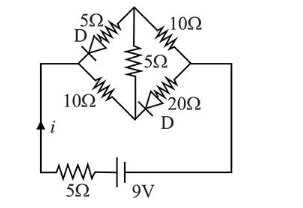# The current i in the network is:Question:

The current $i$ in the network is:1. (1) $0.2 \mathrm{~A}$

2. (2) $0.6 \mathrm{~A}$

3. (3) $0.3 \mathrm{~A}$

4. (4) $0 \mathrm{~A}$

Correct Option: , 3

Solution:

(3) Both the diodes are reverse biased, so, there is no flow of current through $5 \Omega$ and $20 \Omega$ resistances.

Now, two resistors of $10 \Omega$ and two resistors of $5 \Omega$ are in series.

Hence current $I$ through the network

$I=\frac{V}{\mathrm{R}_{\mathrm{eq}}}=\frac{9}{10+5+10+5}$

$\Rightarrow I=\frac{9}{30}=0.3 \mathrm{~A}$